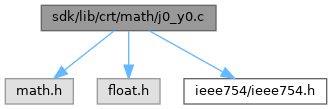ReactOS  0.4.15-dev-3308-g9455def
j0_y0.c File Reference
`#include <math.h>`
`#include <float.h>`
`#include "ieee754/ieee754.h"`
Include dependency graph for j0_y0.c:Go to the source code of this file.

## Functions

int_errno (void)

double _j0 (double num)

double _y0 (double num)

## ◆ _errno()

 int* _errno ( void )

Definition at line 19 of file errno.c.

20 {
22 }
Definition: tls.c:31

Referenced by _j0(), and _y0().

## ◆ _j0()

 double _j0 ( double num )

Definition at line 10 of file j0_y0.c.

11 {
12  if (!_finite(num)) *_errno() = EDOM;
13  return __ieee754_j0(num);
14 }
double __ieee754_j0(double x)
Definition: j0_y0.c:102
#define EDOM
Definition: errno.h:39
_Check_return_ __MINGW_NOTHROW _CRTIMP int __cdecl _finite(_In_ double)
GLuint GLuint num
Definition: glext.h:9618
int * _errno(void)
Definition: errno.c:19

## ◆ _y0()

 double _y0 ( double num )

Definition at line 19 of file j0_y0.c.

20 {
21  double retval;
22  int fpclass = _fpclass(num);
23
24  if (!_finite(num) || fpclass == _FPCLASS_NN ||
25  fpclass == _FPCLASS_ND || fpclass == _FPCLASS_NZ)
26  *_errno() = EDOM;
27
28  retval = __ieee754_y0(num);
29  if (_fpclass(retval) == _FPCLASS_NINF)
30  {
31  *_errno() = EDOM;
32  retval = sqrt(-1);
33  }
34  return retval;
35 }
_STLP_DECLSPEC complex< float > _STLP_CALL sqrt(const complex< float > &)
Definition: complex.cpp:188
#define _FPCLASS_NZ
Definition: float.h:73
#define _FPCLASS_NN
Definition: float.h:71
#define EDOM
Definition: errno.h:39
double __ieee754_y0(double x)
Definition: j0_y0.c:179
_Check_return_ __MINGW_NOTHROW _CRTIMP int __cdecl _finite(_In_ double)
GLuint GLuint num
Definition: glext.h:9618
#define _FPCLASS_ND
Definition: float.h:72
#define _FPCLASS_NINF
Definition: float.h:70
int * _errno(void)
Definition: errno.c:19
_Check_return_ __MINGW_NOTHROW _CRTIMP int __cdecl _fpclass(_In_ double)